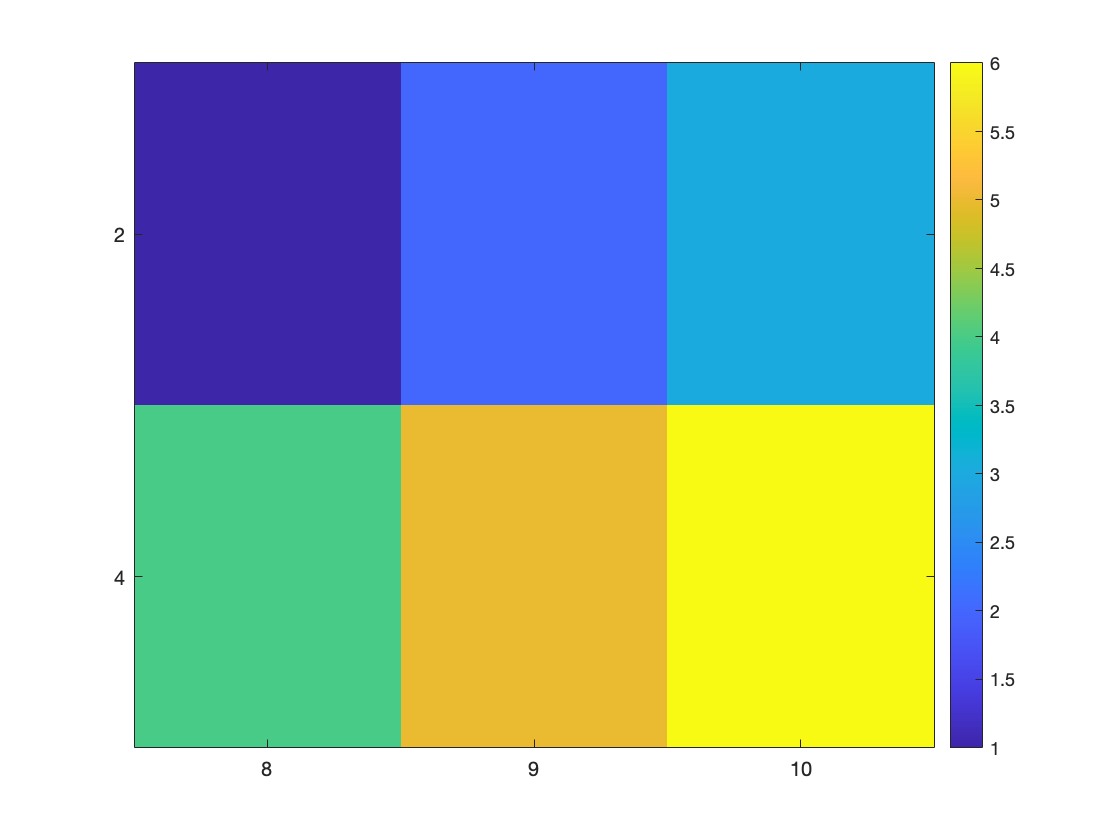# Matlab Concepts

## Cell arrays

Vectors and matrices are great for storing numbers, but what if you want to store other things in an array? One example is if you want to store a bunch of text strings. One way to do this is with a generalization of vectors and matrices called a cell array.
Cell arrays behave similarly (although definitely not identically) to vectors and matrices, but instead of putting numbers into them you can put anything into them. The key difference is instead of using parentheses to index them like vectors and matrices, you use curly brackets { }. For example,
cellArray{1} = 'hi';
cellArray{2} = 120;
cellArray{3} = [1 2 3; 4 5 6];
cellArray
cellArray = 1×3 cell
123
1'hi'120[1,2,3;4,5,6]
Here I made a cell array with a string in the first entry, a number in the second entry and a matrix in the third entry. I can refer back to one of these like this ...
cellArray{3}
ans = 2×3
1 2 3 4 5 6
I can even put cell arrays inside other cell arrays
cellArray2{1,1} = cellArray;
cellArray2{1,2} = 1239;
cellArray2{2,1} = 'hi';
cellArray2{2,2} = [1 2 3];
cellArray2
cellArray2 = 2×2 cell
12
11×3 cell1239
2'hi'[1,2,3]
We've actually already used cell arrays when we made legends for our figures. e.g.
clf;
plot(1:10)
hold on;
plot(10:-1:1)
legend({'line 1' 'line 2'})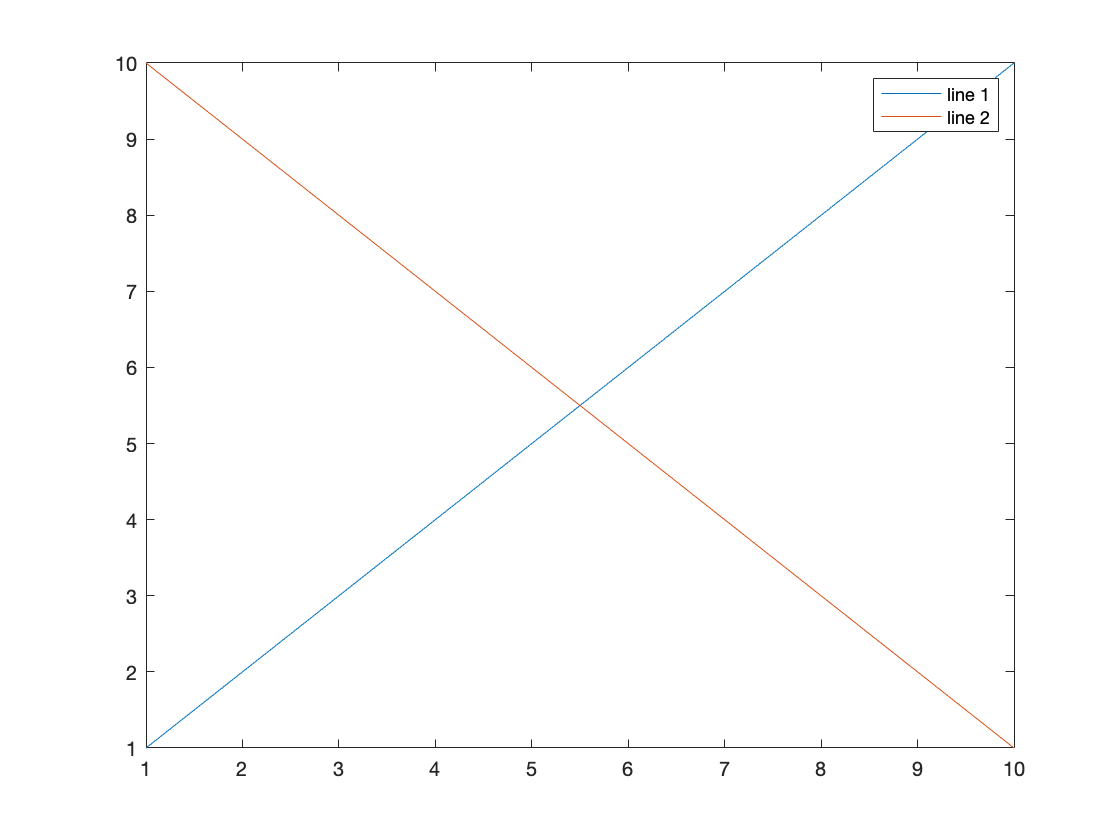Where the input to the legend command is a cell array. We could do the same thing like this ...
clf;
plot(1:10)
hold on;
plot(10:-1:1)
leg{1} = 'line 1';
leg{2} = 'line 2';
legend(leg)## Imaging matrices

Sometimes the easiest way to get a sense of data in a matrix is to image it. Matlab has some functions for this. The simplest is imagesc
M = [1 2 3; 4 5 6];
clf;
imagesc(M)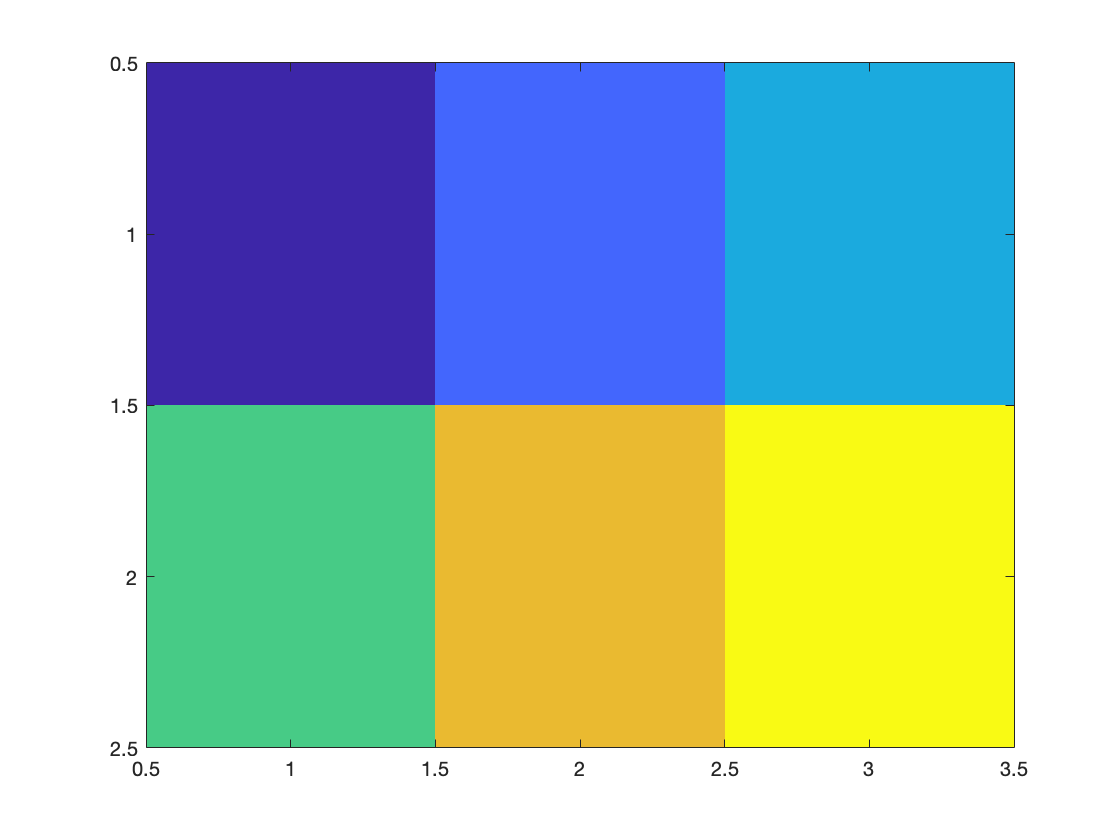If you'd like to know more about what the colors mean you can use the colorbar command ...
clf;
imagesc(M)
colorbar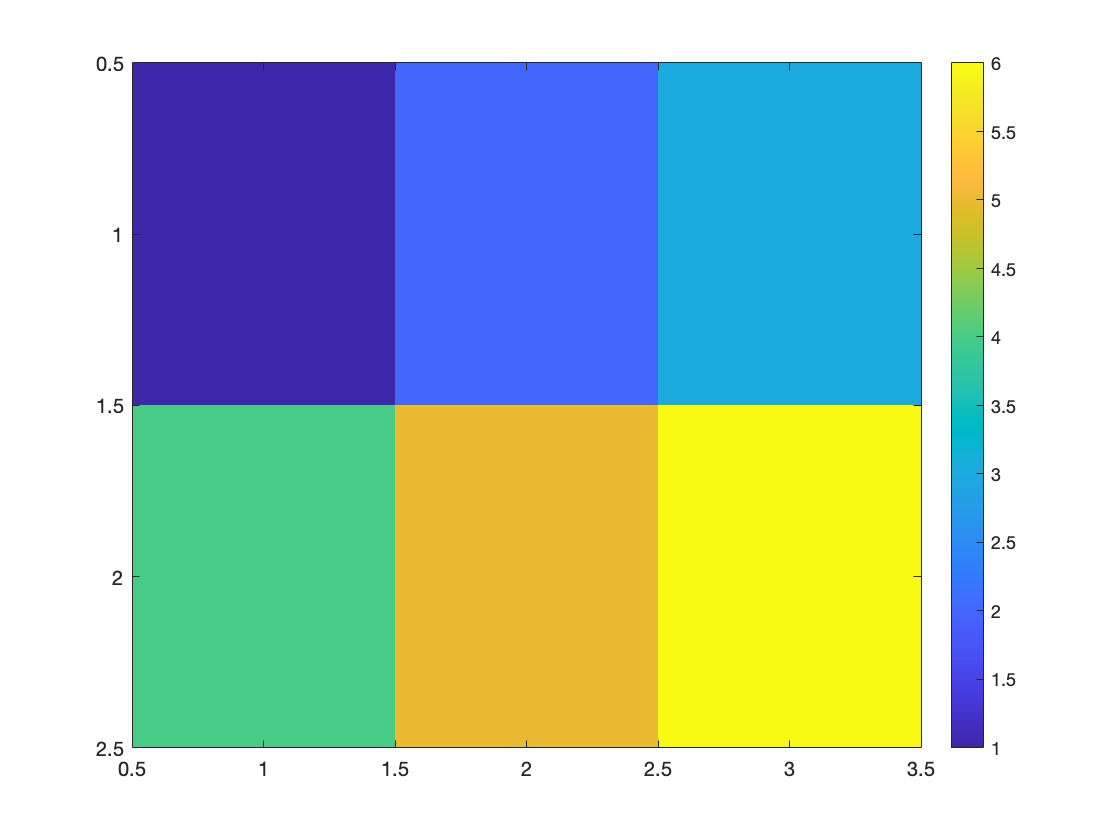which puts a color bar at the side.
Perhaps you'd like to put different numbers on the x or y axis. One way to do that is like this ...
clf;
imagesc([8 9 10], [2 4], M)
colorbar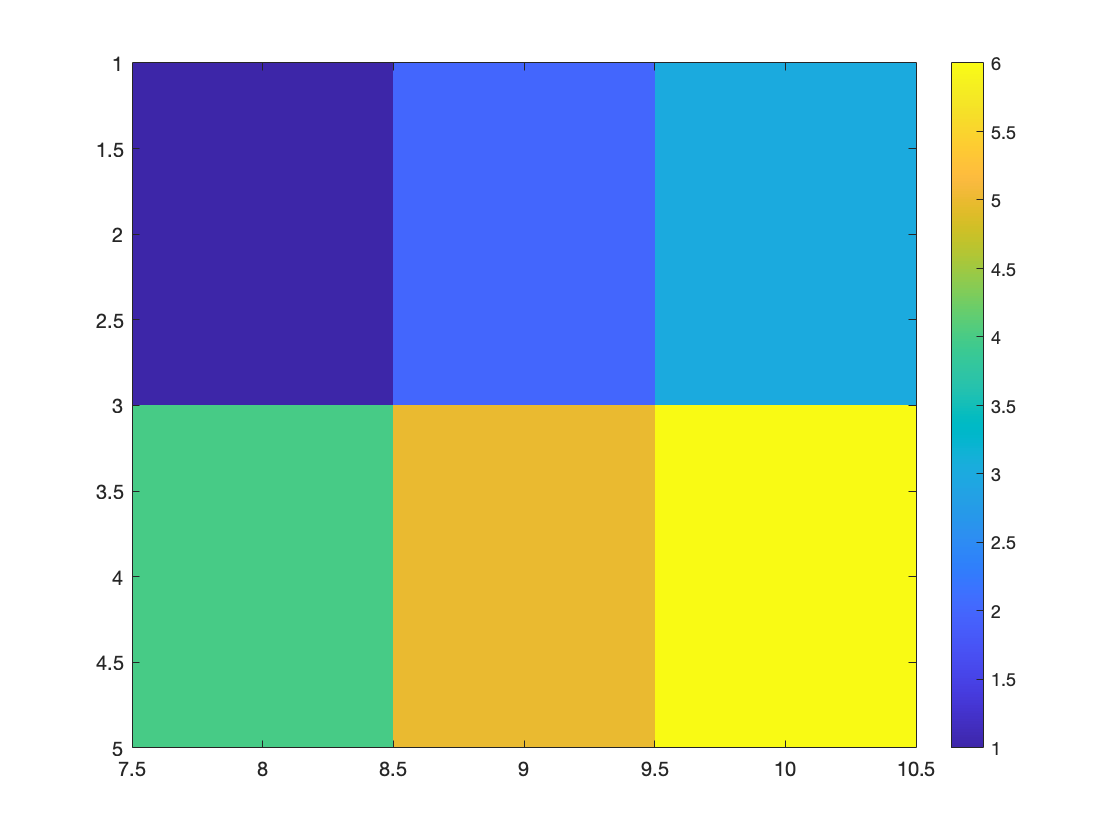Perhaps you'd also like to change where the ticks appear on the x and y axis. You can do this like this ...
clf;
imagesc([8 9 10], [2 4], M)
colorbar
set(gca, 'xtick', [8:10], 'ytick', [2 4])